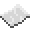# bit32.lshift

Bitwise left-shift. When called, shifts all bits in a number the specified amount to the left. For example, 3 is 11 in binary. Shifting it two bits to the left makes it twelve (1100). A left-shift is also equivalent to number * 2shift.ExampleShifting bits
Shifting ten (1010) 2 bits to the left. This should output 40, as 1010 becomes 101000
Code
```<nowiki>
local ten = 10
print(bit32.lshift(ten, 2))
</nowiki>
```
Output 40

bit32.lshift
Function
Syntax
bit32.lshift(
• number : number
• shift : number
)

Returns number shifted
API bit32
Source Lua (source)